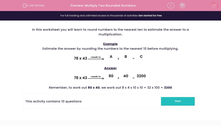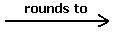# Multiply Two Rounded Numbers

In this worksheet, students use their rounding skills to round numbers to the nearest 10 before multiplying the numbers together.This content is premium and exclusive to EdPlace subscribers.Key stage:  KS 2

Curriculum topic:   Maths and Numerical Reasoning

Curriculum subtopic:   Mixed Problems

Difficulty level:#### Worksheet Overview

In this worksheet you will learn to round numbers to the nearest ten to estimate the answer to a multiplication.

Example

Estimate the answer by rounding the numbers to the nearest 10 before multiplying.

78 x 43A
x
 B
=
 C

78 x 4380
x
 40
=
 3200

Remember, to work out 80 x 40, we work out 8 x 4 x 10 x 10 = 32 x 100 = 3200

### What is EdPlace?

We're your National Curriculum aligned online education content provider helping each child succeed in English, maths and science from year 1 to GCSE. With an EdPlace account you’ll be able to track and measure progress, helping each child achieve their best. We build confidence and attainment by personalising each child’s learning at a level that suits them.

Get started